# Chapter 7, Section 7.7, Question 07 Consider the following system of equations. (a) Find a fundamental matrix for the g...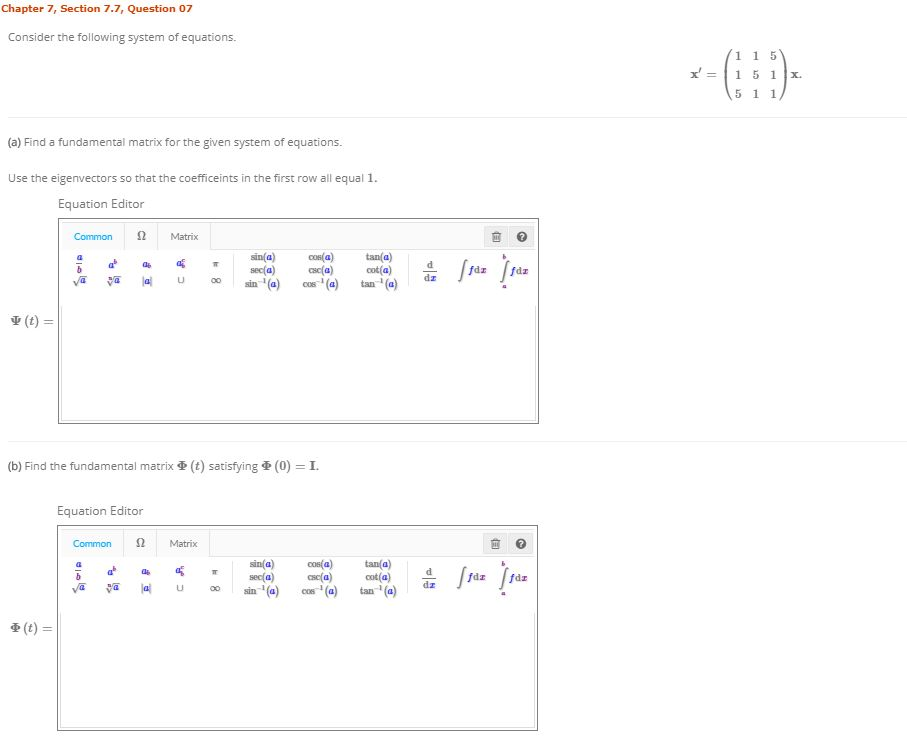Chapter 7, Section 7.7, Question 07 Consider the following system of equations. (a) Find a fundamental matrix for the given system of equations. Use the eigenvectors so that the coefficeints in the first row all equal 1 Equation Editor Ω Common Matrix Ψ (t) = (b) Find the fundamental matrix重(t) satisfying重(0) = 1. Equation Editor Ω Common Matrix tan a) sin(a) 0os(a) 重(t) =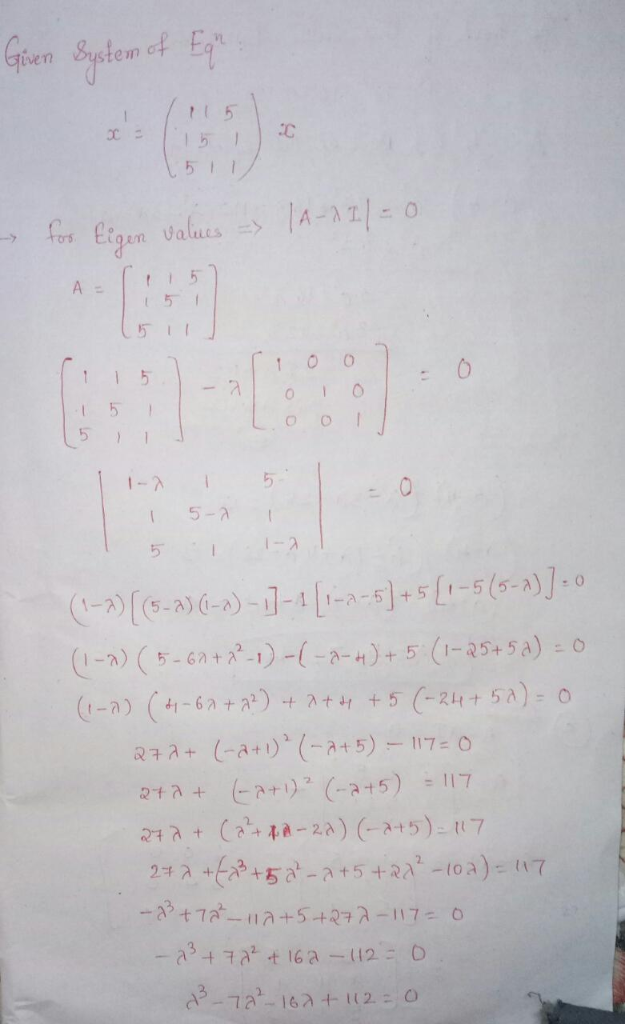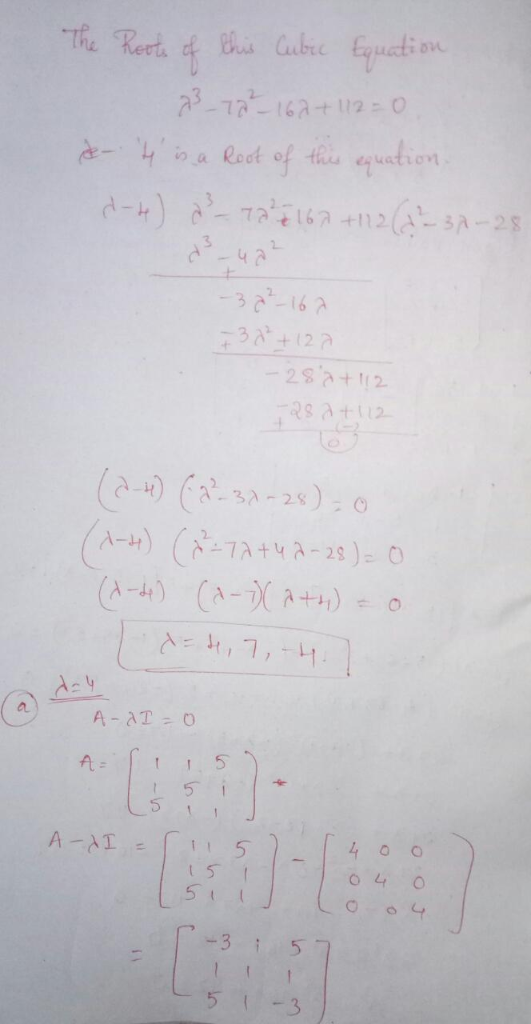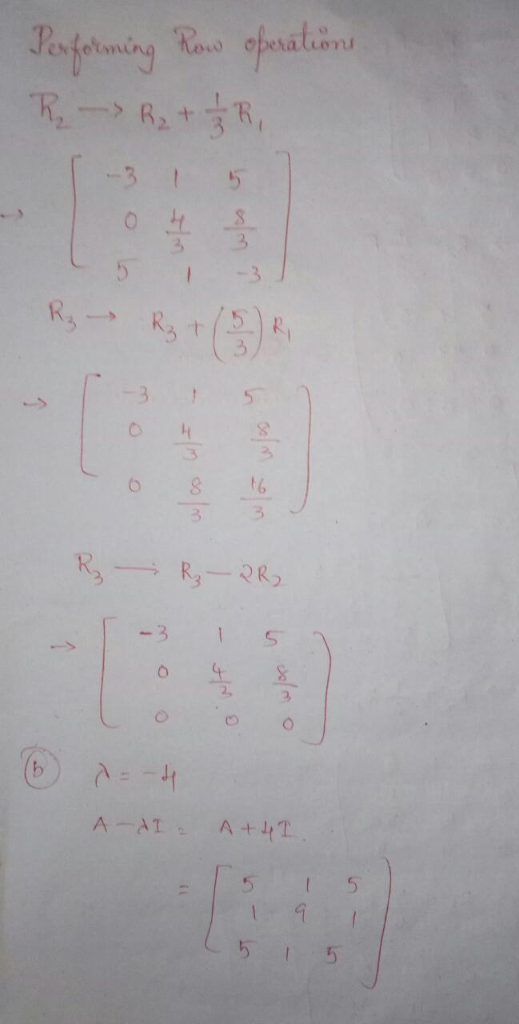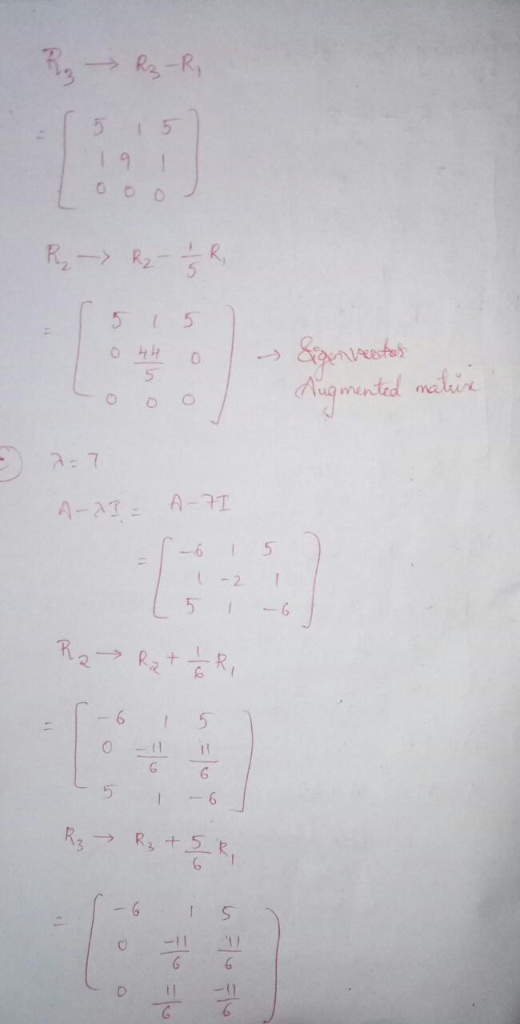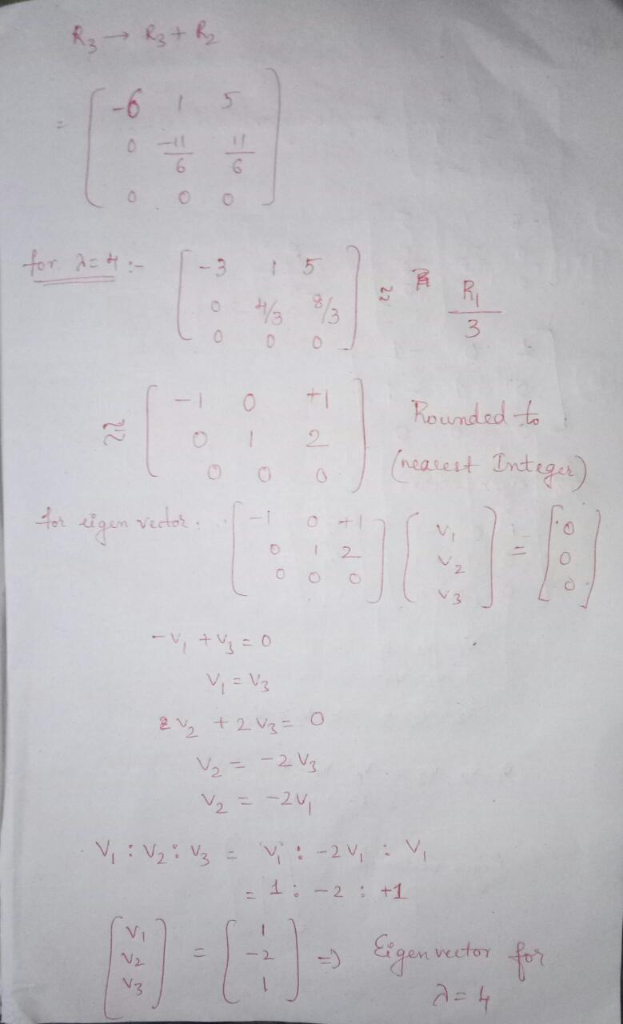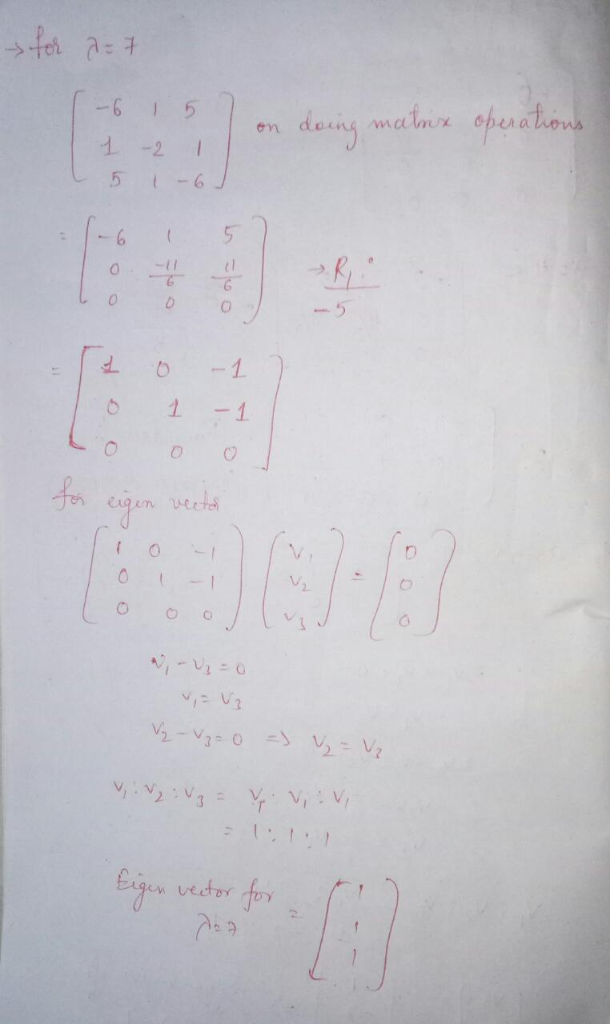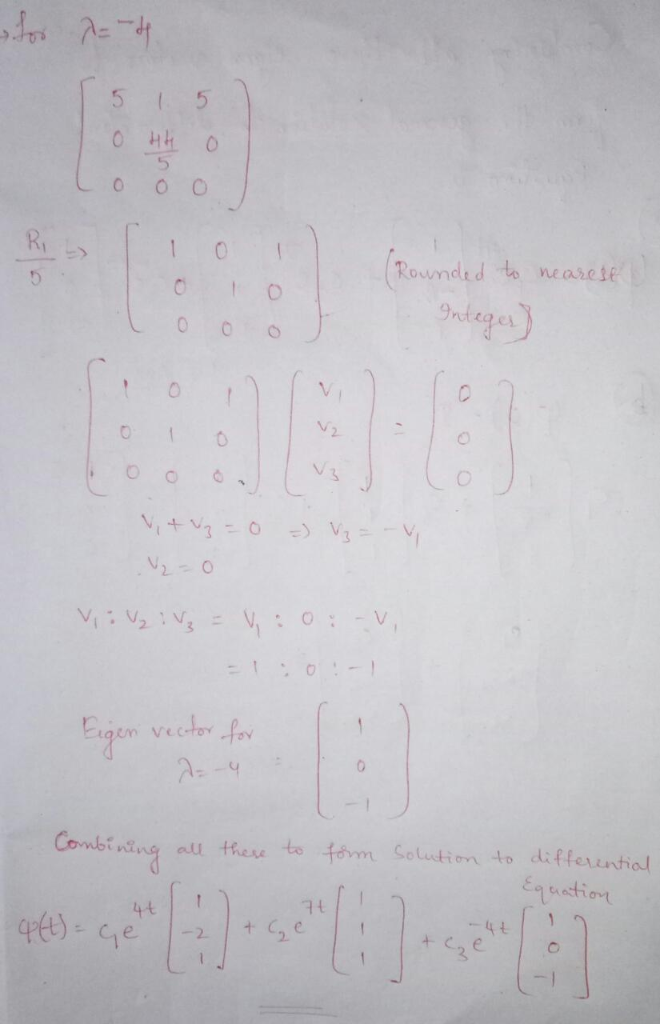i have solved only for a)

##### Add Answer of: Chapter 7, Section 7.7, Question 07 Consider the following system of equations. (a) Find a fundamental matrix for the g...
Similar Homework Help Questions
• ### Chapter 6, Section 6.5, Question 07 Consider the given system of equations. 10-1 (a) Find a funda...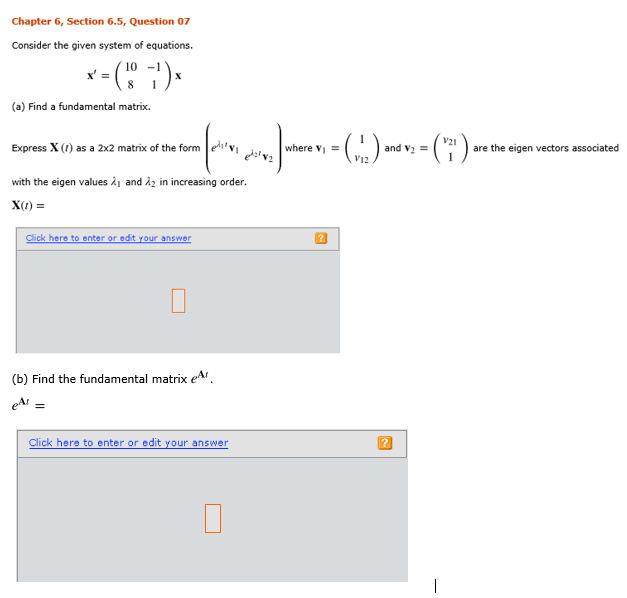Chapter 6, Section 6.5, Question 07 Chapter 6, Section 6.5, Question 07 Consider the given system of equations. 10-1 (a) Find a fundamental matrix. V21 Express X (1) as a 2x2 matrix of the form ei, Vi A. with the eigen values 시 and in increasing order. x(t) = ) and v2 = V12 ) are the eigen vectors associated where v- v e :,v, her (b) Find the fundamental matrix e Ar et Click here to enter or edit...

• ### Consider the vectors x)t) t2 8t and X(2) (t) 10 (a) Compute the Wronskian of x) andx) (b) In wh...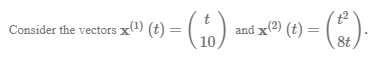Consider the vectors x)t) t2 8t and X(2) (t) 10 (a) Compute the Wronskian of x) andx) (b) In what intervals are x'') and X㈢ linearly independent? Enter the intervals in the ascending order Equation Editor Equation Editor Common Ω Matrix Common Ω Matrix sin(a) sec(a) COR(a) asc(a) cos(a) tan(a) )三三) 읊 //d.fu. tan a sec(a) Equation Editor Equation Editor Common Ω Matrix Common Ω Matrix sec(a) esc(a) oot(a) cse(a) d z e) What conclusion can be drawn about coefficients...

• ### Chapter 6, Section 6.5, Question 06 Consider the given system of equations. (a) Find a fundamenta...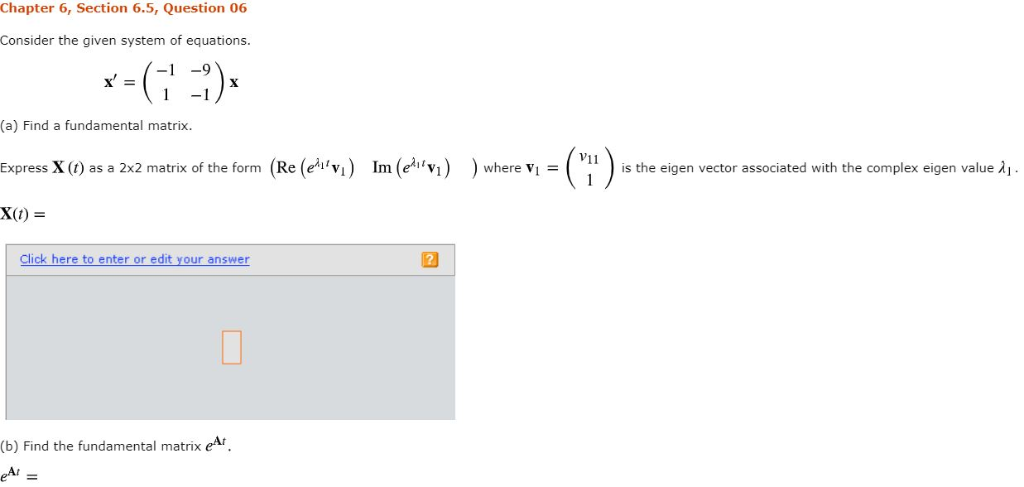Chapter 6, Section 6.5, Question 06 Consider the given system of equations. (a) Find a fundamental matrix Express X (t) as a 2x2 matrix of the form x(t) = where vi-Ci ) s the eigen vector associated with the complex eigen value λί V11 Re (eht vi lm (e,%) Click here to enter or edit your answer (b) Find the fundamental matrix eAr (b) Find the fundamental matrix eAr Click here to enter or edit your answer Click if you...

• ### MESSAGE M Chapter 3, Section 3.6, Question 05 Find the general solution of the differential equat...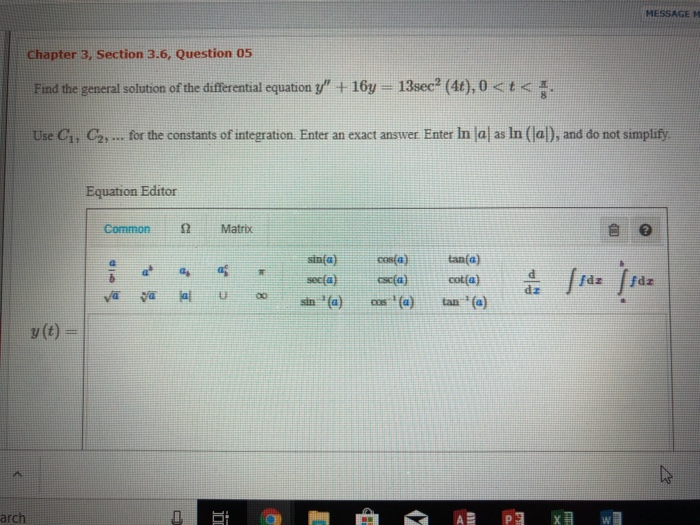MESSAGE M Chapter 3, Section 3.6, Question 05 Find the general solution of the differential equation + 16-13sec"(40, 0 < t <晋 Use C, C2,... for the constants of integration. Enter an exact answer Enter in lal as In (lal), and do not simplify Equation Editor Common Ω Matrix sin(a)cos(a sec(a) 읊 ffdz).dz tan(a) : 떼 y(t)- arch MESSAGE M Chapter 3, Section 3.6, Question 05 Find the general solution of the differential equation + 16-13sec"(40, 0

• ### Consider the following coefficient matrix, which contains a parameter a. 11 6 (a) Determine the e...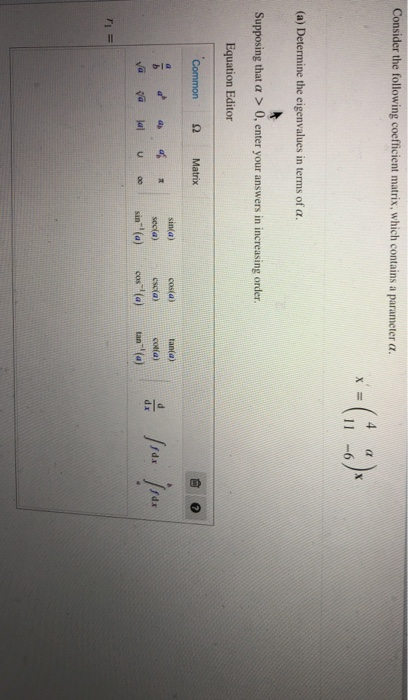Consider the following coefficient matrix, which contains a parameter a. 11 6 (a) Determine the eigenvalues in terms of α. Supposing that α > 0, enter your answers in increasing order. Equation Editor Common Ω Matrix 自0 tania) sin(a) d a 4 secia) esia)a) costa) 邇 alal sin"(a) cos-1(a) tan-"(a)- u oo Ω Matrix cosa) tana) ,..tseela, osia, =a) Va ya lal sin-(a)(a) tan ( o sinia) sec(α) //u),dx ! 읊 cscla) (b) Find the critical value or values of...

• ### Chapter 3, Section 3.2, Question 07 (a) State whether the given system is autonomous or nonautonomous and also whether...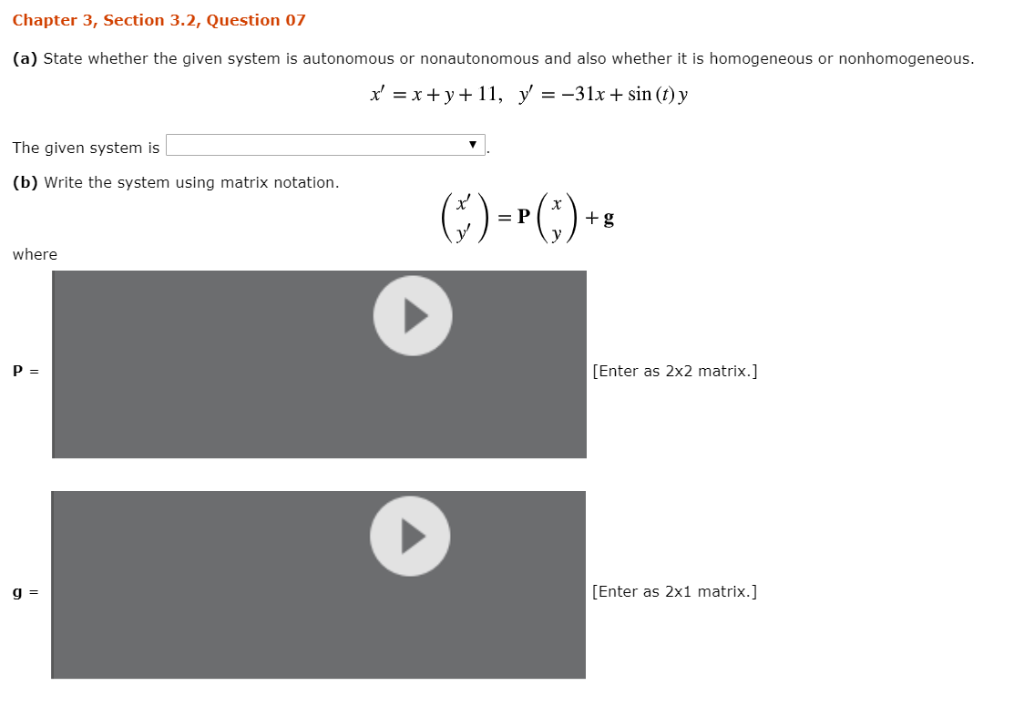Chapter 3, Section 3.2, Question 07 (a) State whether the given system is autonomous or nonautonomous and also whether it is homogeneous or nonhomogeneous. x' x+y11, y = -31x+ sin (t) y The given system is (b) Write the system using matrix notation. ()-) = P +g where [Enter as 2x2 matrix.] P = [Enter as 2x1 matrix. g Chapter 3, Section 3.2, Question 07 (a) State whether the given system is autonomous or nonautonomous and also whether it is...

• ### il Boundary Value Problems, MESSAGE MY INSTRUCTOR STANDARD VIEW Chapter 5, Section 5.3, Question 05 Determine a lower bound for the radius of convergence of series solutions about each given poi...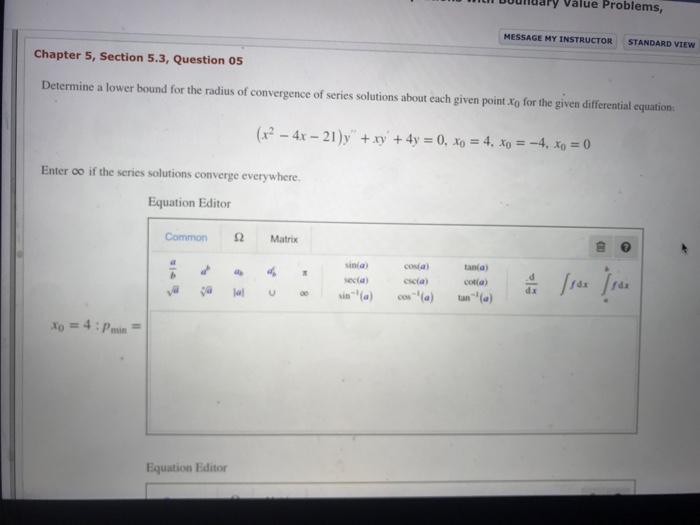il Boundary Value Problems, MESSAGE MY INSTRUCTOR STANDARD VIEW Chapter 5, Section 5.3, Question 05 Determine a lower bound for the radius of convergence of series solutions about each given point Xo for the given differential equation. Enter 00 if the series solutions converge everywhere. Equation Editor Ω Matrix Common sinia secia) sin (a) tania) coia) co a) cos-(a) Equation Editor Equation Editor Common Ω Matrix sinia) secta) scia) =-4 : pmin xo = Equation Editor Ω Matrix Common ina)COa)tania)...

• ### Chapter 3, Section 3.5, Question 15 Find the solution of the initial value problem y" + 2y' + 5У-16e-t cos (2t), y (0)-4, y, (0-0. Enclose arguments of functions in parentheses. For examp...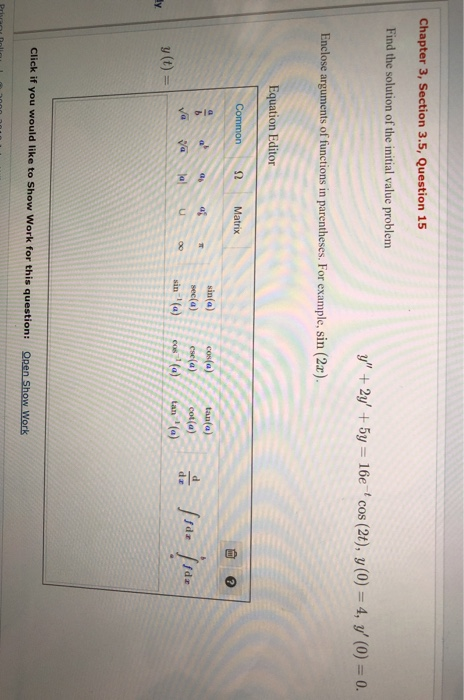Chapter 3, Section 3.5, Question 15 Find the solution of the initial value problem y" + 2y' + 5У-16e-t cos (2t), y (0)-4, y, (0-0. Enclose arguments of functions in parentheses. For example, sin (2x) Equation Editor Ω Common Matrix 亩。 sin(a) ca) tanta) sec(a) ese(a cot(a sin (a) y (t) Click if you would like to Show Work for this question: Open Show Work Chapter 3, Section 3.5, Question 15 Find the solution of the initial value problem y"...

• ### Hi! I need help with this college level differential equations question. Please show all work and...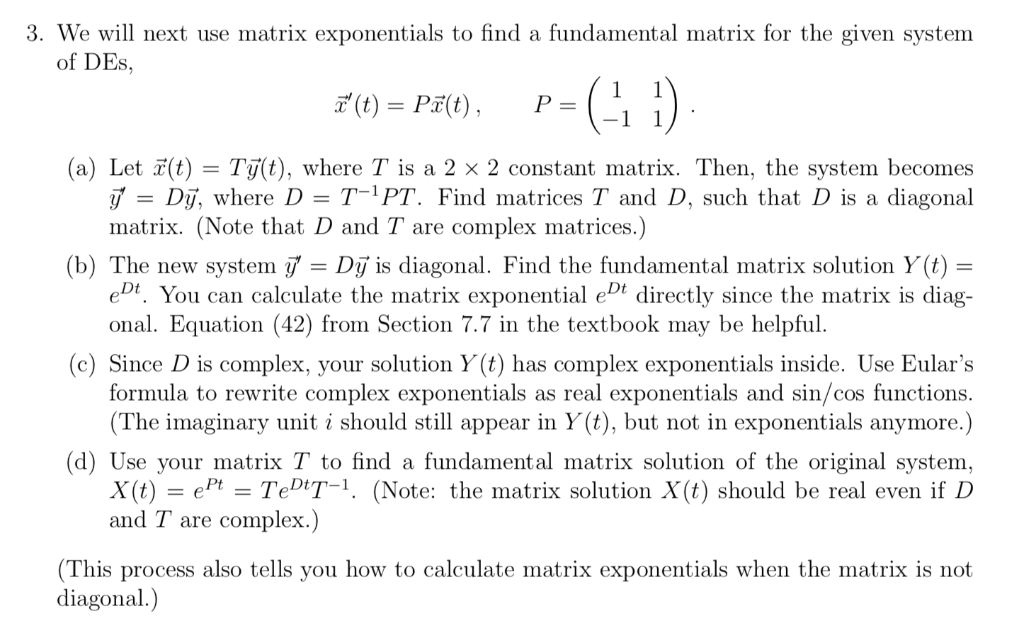hi! I need help with this college level differential equations question. Please show all work and thank you. 3. We will next use matrix exponentials to find a fundamental matrix for the given system of DEs, (t) = P3(t) , P= (a) Letỉ(t) Ty(t), where Ț s a 2 2 constant matrix. Then, the system becomes = Dy, where D-T-1 PT. Find matrices T and D, such that D is a diagonal matrix. (Note that D and T are complex...

• ### Given the following system of linear equations 1. 2xi + 4x2 + 8 x3 + x. +2x,3 a) Write the augmented matrix that represents the system b) Find a reduced row echelon form (RREF) matrix that is row...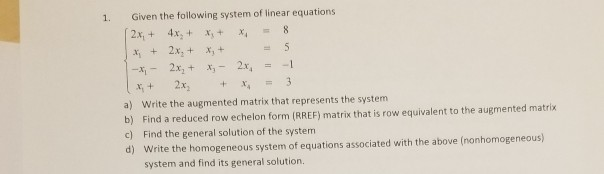Given the following system of linear equations 1. 2xi + 4x2 + 8 x3 + x. +2x,3 a) Write the augmented matrix that represents the system b) Find a reduced row echelon form (RREF) matrix that is row equivalent to the augmented matrix c) Find the general solution of the system d) Write the homogeneous system of equations associated with the above (nonhomogeneous) system and find its general solution. Given the following system of linear equations 1. 2xi + 4x2...

Need Online Homework Help?